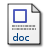# Spreadsheet solution of hyperbolic partial differential equations[for EM field calculations]

Kharab, A. and Kharab, R. (1997) Spreadsheet solution of hyperbolic partial differential equations[for EM field calculations]. Education, IEEE Transactions on, 40.Preview
PDF
17KbMicrosoft Word
26Kb

## Abstract

This paper describes the use of spreadsheet programs for the numerical solution of hyperbolic partial differential equations. A complete 123 macro program is presented and applied to obtain the approximate solution of a model problem in electrical engineering. Through user macros, spreadsheets can now function in the same way as programs written in conventional programming languages. They provide a clear and direct means of entering data and formulas. This important feature will allow the students to easily experiment with their model problems

Item Type: Article February 1997 Publication Computer College Of Sciences > Mathematical Science Dept Kharab, A. and Kharab, R. 14332 KFUPM ePrints Admin 24 Jun 2008 16:32 12 Apr 2011 13:15

Repository Staff Only: item control page